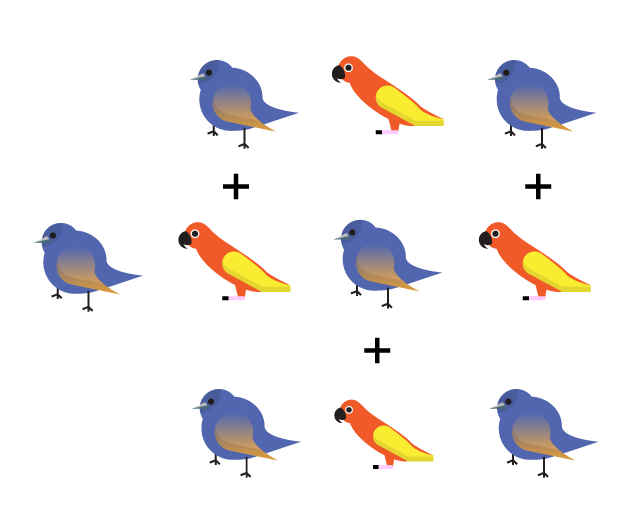# Sums Of 10

Probability Level 3How many ways are there of adding together two or more integers from $1$ to $9$ to make $10$? For example, $1+9=10$, $9+1=10$, $2+8=10$, $8+2=10$, and $2+1+2+1+1+2+1=10$. (Sums that contain the same numbers but in a different order are considered to be different.)

×

Problem Loading...

Note Loading...

Set Loading...